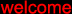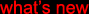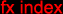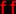(c) 2000-2009

mode zero

mu-tron bi-phase

True stereo mode (six stages on each channel).

// = A/B (left/right) settings, otherwise both channels are set identically.

The settings used for "drums B, #2" were lost, sorry!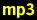drums B, no effectdrums B, rate = 2 // 2.5, depth = 5, feedback = max, sine, sync = revdrums B, #2guitar, no effectguitar, rate = near 2, depth = max, feedback = max, sine, sync = revguitar, rate = near 2, depth = max, feedback = min, sine, sync = revguitar, rate = near 7, depth = 5 // 6, feedback = 5, sine, sync = revrhythm guitar A, no effectrhythm guitar A, rate = 6.5 // 5.5, depth = max, feedback = max, sine, sync = revrhythm guitar A, rate = 2 // 2.5, depth = 5, feedback = 5, sine, sync = revrs09 strings, no effectrs09 strings, rate = 4 // 2, depth = 7 // 10, feedback = 0 // 10, sine, sync = revrs09 strings, rate = 6 // 4.5, depth = max, feedback = max, square, sync = revrs09 strings, rate = 2 // 2.5, depth = max, feedback = max, square, sync = revmatrix-1000 pad, no effectmatrix-1000 pad, rate = 2.5 // 3, depth = max, feedback = max, sine, sync = revmatrix-1000 pad, rate = 7 // 2, depth = 10 // 7, feedback = 2.5 // 10, sine, sync = rev

A-into-B quasi 12 stage mode.

// = A/B settings, otherwise both channels are set identically.  See above for dry samples.rs09 strings, rate = 2 // 7, depth = 10 // 5, feedback = 10, sine, sync = normrs09 strings, rate = 2 // 8, depth = 10, feedback = 10, sine // square, sync = normrs09 strings, rate = near 3, depth = 5.5, feedback = 5, sine, sync = normguitar, rate = 3 // 3.5, depth = 10, feedback = 0, sine, sync = normguitar, rate = 2 // 7, depth = 5 // 6, feedback = 0 // 10, sine, sync = normguitar, rate = 2 // 7, depth = 5 // 6, feedback = 10 // 0, sine, sync = normguitar, rate = 2 // 7, depth = 10 // 5, feedback = 10, sine, sync = norm